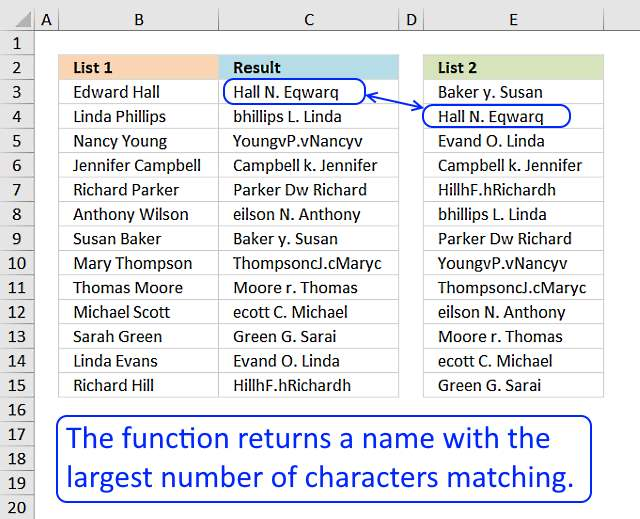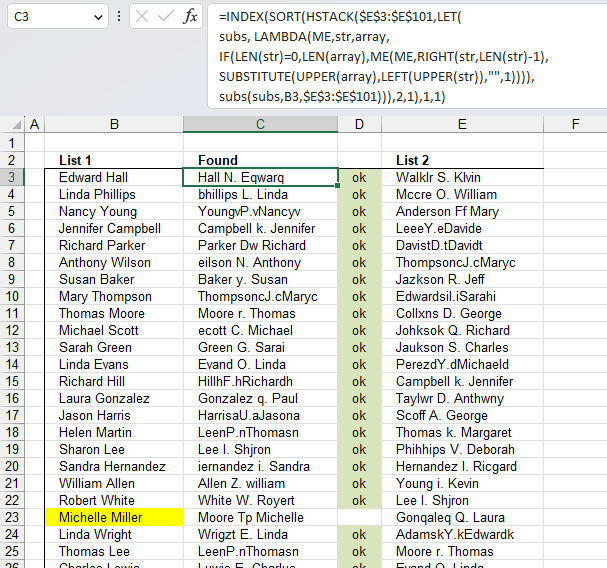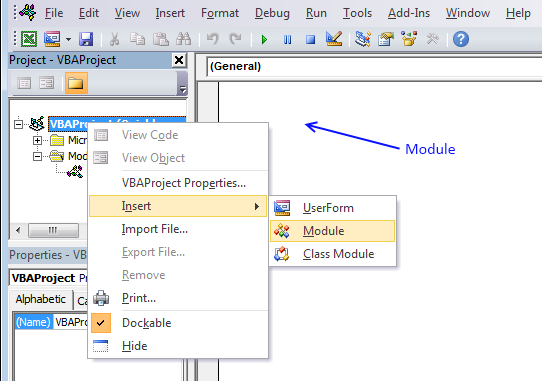Author: Oscar Cronquist Article last updated on April 03, 2023In this post I will describe a basic user defined function with better search functionality than the array formula in this post: Fuzzy vlookup. I will also demonstrate a recursive LAMBDA function that does the exact thing as the UDF.

Here is what they do:

1. Iterate through each character.
2. Check if the character exists.
3. Count the number of characters that match (not case sensitive).
4. Return the value from List 2 that has the most number of matches.

## 1. Fuzzy lookups - Excel 365 recursive LAMBDA functionThe following formula compares a value in one cell to values in a cell range character by character. It keeps counting matching characters and the value with most matching characters is returned.

The example in the image above shows "Edward Hall" in cell B3, the formula in cell C3 compares the value in cell B3 to all values in cell range E3:E101 and returns the value from E3:E101 with the highest matching character count. The formula returns "Hall N. Eqwarq ", the names seems to be misspelled and the last name is first and then the first name.

This is useful if you want to compare two columns and there are missing characters or strings are rearranged in a cell.

Excel 365 recursive LAMBDA function in cell C3:

=INDEX(SORT(HSTACK(\$E\$3:\$E\$101,LET(
subs, LAMBDA(ME,str,array,
IF(LEN(str)=0,LEN(array),ME(ME,RIGHT(str,LEN(str)-1),SUBSTITUTE(UPPER(array),LEFT(UPPER(str)),"",1)))),
subs(subs,B3,\$E\$3:\$E\$101))),2,1),1,1)

Copy cell C3 and paste to cells below as far as needed.

Adjust cell references B3 and \$E\$3:\$E\$101 in the formula above so they work with your worksheet, that is all.

### Explaining formula

#### Step 1 - Convert letters to upper letters

The UPPER function converts a value to upper case letters.

Function syntax: UPPER(text)

UPPER(str)

#### Step 2 - Extract first character from the left

The LEFT function extracts a specific number of characters always starting from the left.

Function syntax: LEFT(text, [num_chars])

LEFT(UPPER(str))

#### Step 3 - Substitute character with noting "" and only the first instance

The SUBSTITUTE function replaces a specific text string in a value. Case sensitive.

Function syntax: SUBSTITUTE(text, old_text, new_text, [instance_num])

SUBSTITUTE(UPPER(array),LEFT(UPPER(str)),"",1)

#### Step 4 - Count characters

The LEN function returns the number of characters in a cell value.

Function syntax: LEN(text)

LEN(str)

#### Step 5 - Remove first character

The RIGHT function extracts a specific number of characters always starting from the right.

Function syntax: RIGHT(text,[num_chars])

RIGHT(str, LEN(str)-1)

#### Step 6 - Create recursive function named ME

Some prefer using the ME combined with the LET function while building and troubleshooting the LAMBDA formula. Then create a named formula in the Name Manager, however, I am not going to use the Name manager at all in this example.

ME(ME,RIGHT(str,LEN(str)-1),SUBSTITUTE(UPPER(array),LEFT(UPPER(str)),"",1))

#### Step 7 - Loop until string is empty

The IF function returns one value if the logical test is TRUE and another value if the logical test is FALSE.

Function syntax: IF(logical_test, [value_if_true], [value_if_false])

The IF function allows us to iterate through each character until there are no characters left.

IF(LEN(str)=0,LEN(array),ME(ME,RIGHT(str,LEN(str)-1),SUBSTITUTE(UPPER(array),LEFT(UPPER(str)),"",1)))

The logical_test is LEN(str)=0, if this returns TRUE then the second argument is run, if FALSE then the third argument. The IF function controls the entire recursive function.

logical_test : LEN(str)=0
[value_if_true] - LEN(array)
[value_if_false] - ME(ME, RIGHT(str, LEN(str)-1), SUBSTITUTE(UPPER(array), LEFT(UPPER(str)), "", 1))

[value_if_false] is repeated until the string str is empty. Then the IF function returns the character count using the LEN function. LEN(array)

Excel lets you populate the array variable with multiple values from a cell range, this creates an algorithm that calculates all values in \$E\$3:\$E\$101 simultaneously.

#### Step 8 - Build LAMBDA function

The LAMBDA function build custom functions without VBA, macros or javascript.

Function syntax: LAMBDA([parameter1, parameter2, …,] calculation)

The LAMBDA function lets you name parameters and specify a formula.

LAMBDA(ME,str,array,
IF(LEN(str)=0,LEN(array),ME(ME,RIGHT(str,LEN(str)-1),SUBSTITUTE(UPPER(array),LEFT(UPPER(str)),"",1))))

#### Step 9 - Name LAMBDA function

The LET function lets you name intermediate calculation results which can shorten formulas considerably and improve performance.

Function syntax: LET(name1, name_value1, calculation_or_name2, [name_value2, calculation_or_name3...])

I am naming the LAMBDA function subs.

LET(
subs, LAMBDA(ME,str,array,
IF(LEN(str)=0,LEN(array),ME(ME,RIGHT(str,LEN(str)-1),SUBSTITUTE(UPPER(array),LEFT(UPPER(str)),"",1)))),
subs(subs,B3,\$E\$3:\$E\$101))

#### Step 10 - Add arrays horizontally

The HSTACK function combines cell ranges or arrays. Joins data to the first blank cell to the right of a cell range or array (horizontal stacking)

Function syntax:

HSTACK(\$E\$3:\$E\$101,LET(
subs, LAMBDA(ME,str,array,
IF(LEN(str)=0,LEN(array),ME(ME,RIGHT(str,LEN(str)-1),SUBSTITUTE(UPPER(array),LEFT(UPPER(str)),"",1)))),
subs(subs,B3,\$E\$3:\$E\$101)))

#### Step 11 - Sort array by the second column

The SORT function sorts values from a cell range or array

Function syntax: SORT(array,[sort_index],[sort_order],[by_col])

Sort the array based on the second column which contains the character count.

SORT(HSTACK(\$E\$3:\$E\$101,LET(
subs, LAMBDA(ME,str,array,
IF(LEN(str)=0,LEN(array),ME(ME,RIGHT(str,LEN(str)-1),SUBSTITUTE(UPPER(array),LEFT(UPPER(str)),"",1)))),
subs(subs,B3,\$E\$3:\$E\$101))),2,1)

#### Step 12 - Get value

The INDEX function returns a value or reference from a cell range or array, you specify which value based on a row and column number.

Function syntax: INDEX(array, [row_num], [column_num])

Get the top left value in the sorted array.

INDEX(SORT(HSTACK(\$E\$3:\$E\$101,LET(
subs, LAMBDA(ME,str,array,
IF(LEN(str)=0,LEN(array),ME(ME,RIGHT(str,LEN(str)-1),SUBSTITUTE(UPPER(array),LEFT(UPPER(str)),"",1)))),
subs(subs,B3,\$E\$3:\$E\$101))),2,1),1,1)

### Get the Excel fileSearch_characters-Excel-365-recursive-LAMBDA-function.xlsx

## 2. Fuzzy lookups [UDF]The user defined function searches for a value with as many characters matching as possible. It is as simple as that.

I have added some serious misspellings randomly in List 2. 98 out of 100 names are found.

### User defined Function Syntax

SEARCHCHARS(lookup_value, tbl)

### Arguments

 lookup_value Required. The value you want to lookup. [tbl] Required. The range you want to search.

### Example

User defined function in cell C3:

=SEARCHCHARS(B3, \$E\$3:\$E\$101)

Copy cell C3 and paste it to the cells below, as far as needed.

### Excel vba

```'Name function and arguments
Function SearchChars(lookup_value As String, tbl_array As Range) As String
'Declare variables and types
Dim i As Integer, str As String, Value As String
Dim a As Integer, b As Integer, cell As Variant
'Iterste through each cell
For Each cell In tbl_array
'Save cell value to variable
str = cell
'Iterate through characters
For i = 1 To Len(lookup_value)
'Same character?
If InStr(cell, Mid(lookup_value, i, 1)) > 0 Then
'Add 1 to number in array
a = a + 1
'Remove evaluated character from cell and contine with remaning characters
cell = Mid(cell, 1, InStr(cell, Mid(lookup_value, i, 1)) - 1) & Mid(cell, InStr(cell, Mid(lookup_value, i, 1)) + 1, 9999)
End If
'Next character
Next i

a = a - Len(cell)
'Save value if there are more matching characters than before
If a > b Then
b = a
Value = str
End If

a = 0
Next cell
'Return value with the most matching characters
SearchChars = Value
End Function
```